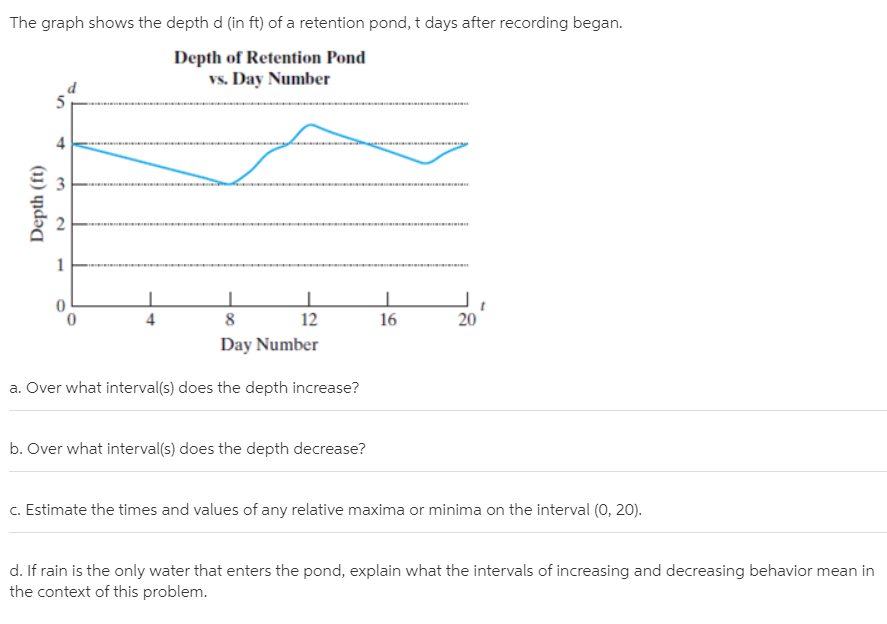# The graph shows the depth d (in ft) of a retention pond, t days after recording began. Depth of Retention Pond vs. Day Number 4 4 12 16 20 Day Number a. Over what interval(s) does the depth increase? b. Over what interval(s) does the depth decrease? c. Estimate the times and values of any relative maxima or minima on the interval (0, 20). d. If rain is the only water that enters the pond, explain what the intervals of increasing and decreasing behavior mean in the context of this problem. 3. 2. Depth (ft)

Questionhelp_outlineImage TranscriptioncloseThe graph shows the depth d (in ft) of a retention pond, t days after recording began. Depth of Retention Pond vs. Day Number 4 4 12 16 20 Day Number a. Over what interval(s) does the depth increase? b. Over what interval(s) does the depth decrease? c. Estimate the times and values of any relative maxima or minima on the interval (0, 20). d. If rain is the only water that enters the pond, explain what the intervals of increasing and decreasing behavior mean in the context of this problem. 3. 2. Depth (ft) fullscreen